• GA_shirtgru_矩形排样_矩形件排样_矩形排样算法_遗传算法矩形.zip
• GA_shirtgru_矩形排样_矩形件排样_矩形排样算法_遗传算法矩形_源码.zip
• TypeSet类， 在界面中显示矩形排样结果，VS2008 运行
• 在java编程环境下基于遗传算法的生成矩形排样
• 看了网上好多文章讲矩形排样，自己也想了很多。总结了一下： 1，矩形如何排列 目标矩形的1,2,3,4脚点可能分别排列3个不与目标矩形重叠的3个矩形。 2，判断待排列矩形与画面中其他矩形的关系 2.1 是否...

看了网上好多文章讲矩形排样，自己也想了很多。总结了一下：

1，矩形如何排列目标矩形的1,2,3,4脚点可能分别排列3个不与目标矩形重叠的3个矩形。

2，判断待排列矩形与画面中其他矩形的关系
2.1 是否超出画面大小
2.2 是否与画面内其他矩形重叠
3， 取最优解
模拟退火算法，遗传算法，可获得最优解

算法优化：
适合的解数量太多，导致程序执行效率不高。
准备增加线程的方式，来提高执行效率。

转载于:https://www.cnblogs.com/new0801/archive/2011/04/02/6175995.html
展开全文• 矩形排样在有广泛的应用,目标是使下料过程中的切割损失最小,使得原材料的利用率最高.对矩形排样进行实际问题的求解。 来自https://github.com/KevAli/RectangleLayoutCppCode
• 矩形排样的一种人机结合的 优化算法方式描述 针对当前的矩形排样优化问题
• 利用遗传算法解决矩形排样问题，具有可视化的界面，输入数据为含有矩形的长和宽的文本文件，输出的结果以可视化的形式显示出来
• C++ 基于遗传算法的矩形排样 具有可视化的界面。 利用遗传算法解决矩形排样问题，具有可视化的界面，输入数据为含有矩形的长和宽的文本文件，输出的结果以可视化的形式显示出来 MFC 遗传算法 矩形排样
• 二维矩形排样相关论文，是参加数学建模比赛用到的相关论文。
• 优化排样问题的近似算法优化排样问题的近似算法矩形排样C++例子
• ## 关于矩形排样问题(三)

万次阅读 多人点赞 2015-07-11 01:41:24
上一篇博客 关于矩形排样问题(二) 给出了矩形排样问题的背景，并对遗传算法做出了详细的介绍。但是没有给出具体的解决方法，这里针对矩形排样问题，给出基于遗传算法的解法。利用率的定义谈到矩形排样问题，必然涉及...
上一篇博客 关于矩形排样问题(二) 给出了矩形排样问题的背景，并对遗传算法做出了详细的介绍。但是没有给出具体的解决方法，这里针对矩形排样问题，给出基于遗传算法的解法。
利用率的定义
谈到矩形排样问题，必然涉及到板材的利用率问题，这里的利用率定义如下：  假设n种小矩形Ri的面积分别为Si(i=1,2,…,n)，数量分别为ni(i=1,2,…,n)，宽为wi，高为li，以 (wi,li)表示，则要下料的矩形毛坯总数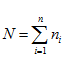，设原料板材宽为 W，高为L ，以 (W,L)表示，矩形件优化排样的目标是，找到一种下料这个矩形毛坯的方法，使得“一刀切”后余料面积 尽可能大，以使得余料可以用来继续加工成可用的新零件，如下图所示，这样达到板材利用率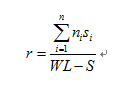尽可能大。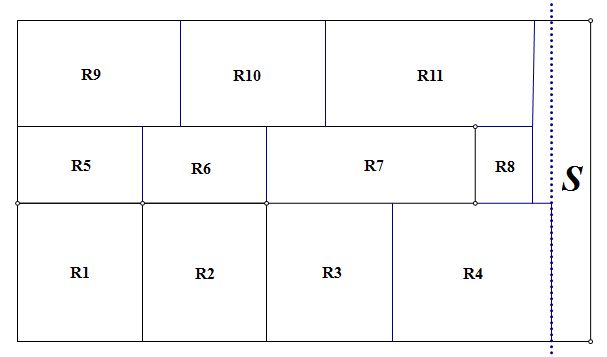布局规则
还要考虑的一个问题就是布局规则，主要有以下几种方式：定位规则、组合规则和邻接规则。
定位规则 待排矩形件零件编号确定后，接下来的工作就是确定被选待排炬形件在布局  空间中的摆放位置，总体有以下3种：  ①占角策略，即将待排矩形件摆放在布局空间的某一角；  ②顺放策略，即从布局空间的某一角开始，将待排矩形件沿着布局空间的  某一边摆放；  ③在底盘装载问题中，先将待排矩形件沿布局空间的四边放置，最后摆放  布局空间的中心。  考虑到钢结构排样的实际约束条件，本文采用的是第1种先占左下角的定位规则。组合规则  为提高工业生产的加工效率，在设计布局方案时应考虑组合矩形。组合矩形是由相同长宽的矩形件以同一种排放方式顺次排在同一层上而形成的。组合矩形的4种情况，如下图所示：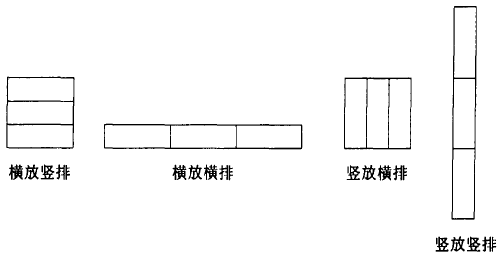邻接规则  领接规则是指将长宽相同或相近的矩形件放在一起进行排料。  规则1：将长或宽相同的矩形相邻排放.规则1的3种情况如下图所示。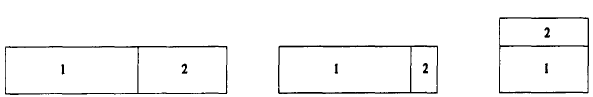规则2：将长或宽相近的矩形相邻排放.规则2的3种情况如下图所示。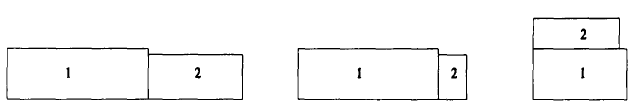一般在排样过程即将结束，当（剩余矩形总面积）/（板材面积）小于一定比例时采用领接规则。尤其对于最后一块板材的排料，采用领接规则能更进一步地提高排样布局的合理性，减少材料浪费。
解决方案
对于矩形排样问题，一个简单的方法就是根据各种长度限制，列出相应的公式，进行暴力搜索，但这种方法的时间复杂度较高，在计算量小的情况下还可以接受，但是在实际的工程应用中用处不大。这里利用最低轮廓线的思想，其核心想法是采用最佳匹配搜索策略来确定矩形件的最佳排放位置，即搜索最低轮廓线中轮廓线的长度与待排矩形件的宽度差值最小的一条轮廓线，直至所有矩形件排样完毕。为了满足实际工程中“一刀切”的要求，需要对其进行修改，这里结合分层的思想。分层排样的思想体现的是一种剪切排样的方式，满足“一刀切”的工艺约束，它是将矩形零件按一定的顺序排放，在排放下一零件时若当前层剩余空间不足，则以当前层所排矩形零件的最高水平线为基准开辟新层。传统的分层排样方式是用水平线将板材分成多层的，排样方式如下图所示：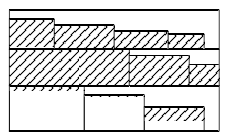下面进入解决问题的正题：结合遗传算法解决排料问题。采用遗传算法对一刀切矩形排料问题进行求解，在排料过程中引入了改进的最低轮廓线算法思想。上一篇博客对遗传算法进行了详细的介绍，这里不再赘述。总结来说，GA仿照染色体的基因在进化的过程中进行选择交叉变异生成下一代种群。 计算开始时对种群进行初始化，并计算每一个个体的适应度函数，生成新的一代。如果生成的种群不满足优化条件，则按照适应度选择个体，父代进行交叉或变异生成子代，然后子代取代父代，再生成下一个子代。这一过程循环执行，直到满足优化准则为止。下面给出各个步骤的具体实施方案：  问题编码：  个体编码是n 个小矩形Ri 的下标 1,2,3,…,n的一个排列如下图所示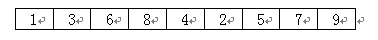初始种群：  设种群中个体的数量为P_SIZE ,随机产生 P_SIZE个个体Xi，Xi 的基因序列是 1,2,3,…,n的一个排列。  定义适应值函数：  对于个体Xi 的基因序列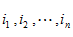对应 n个小矩形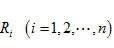的一个排列：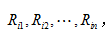按照上述的排料方法, 依次布局在板材上，得到板材的利用率r(i) 。显然板材的利用率r(i) 越大，个体 Xi对应的布局就越优。 因此定义个体的适应值函数为：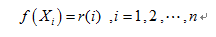遗传选择操作：  计算个体的生存概率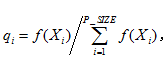在父代种群中按轮盘赌的选择方式选择生存概率大的个体进入子代。  遗传交叉操作：  以交叉概率 Pc选择 2个个体 Xi和 Xj进行交叉操作。随机选择一个交叉点，在交叉点处，交换 2个个体后半部分得到 2个新的个体Xi’ 和 Xj’, 如下图所示：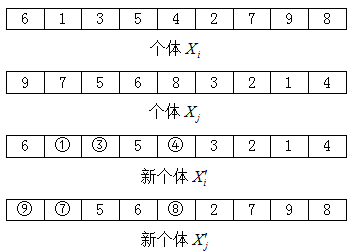很显然，交叉操作可能产生非法个体，即个体中有重复的基因。所以必须对 2个新个体的基因进行调整。
第1步：找出个体Xi’ 的重复基因1、2、4，个体 Xj’的重复基因9、7、8；第2步：将1、3、4与9、7、8对应交换，得到了2个合法的新个体Xi” 和 Xj” 如下图所示：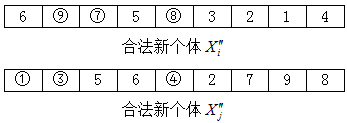遗传变异操作：  以变异概率Pm 选择某一个体进行变异操作。若按普通的变异操作也会产生不合法的个体，所以要设计一种新的变异算子。第1步：在要进行变异的个体中，随机选择2个基因位；第2步：将所选择 2个基因位上的基因值交换，得到 1个新个体。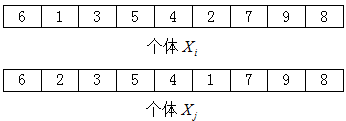在每代的遗传操作过程中，保存最优个体进入子代，这样至少使子代不会比父代差。同时加入一些新品种，即随机产生一些新个体替换老个体，以防止陷入僵局。整个遗传算法的流程图如下图所示：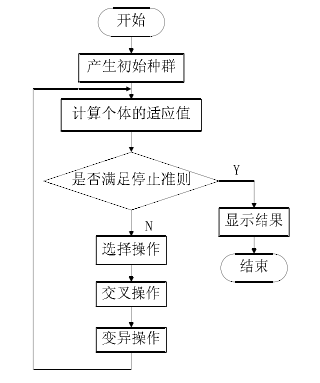编码实现
下面给出具体的编码实现。对于遗传算法的实现网上有一大堆解法，编程语言也各式各样，典型的编程工具包是MATLAB，研究得很透彻，很成熟，已经封装成函数，直接调用传入相应的参数就可以得到结果。这里给出基于VC的编码方法。  整个窗体设计比较简陋，几个Button和文本编辑框以及图形控件用来显示排样的结果。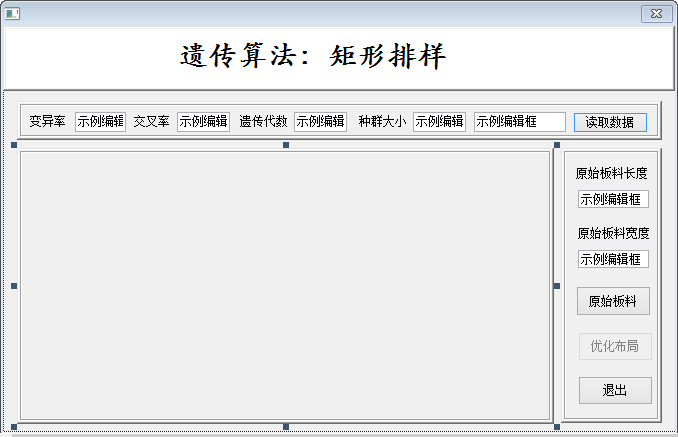下面只给出核心部分的实现(程序写得比较粗糙，只实现了算法，细节的部分还待完善，呵呵)。  首先在主窗体中声明表示个体的结构体：
typedef struct//针对遗传算法定义的一个个体
{
int gene;
double fitness;
double rfitness;
double cfitness;
}GenoType;
以及作为原材料的矩形板材：
typedef struct //矩形板
{
int l;
int w;
int x;
int y; //矩形的右下角
int flag;
}RectAA;

typedef struct //矩形板
{
int l;
int w;
int x;
int y;//矩形的左上角
}RectBB;
考虑到算法实现的可视化，中间大部分区域用于显示排样的结果，以彩色矩形块的方式显示出来，实现比较简单，不是重点。  下面是遗传算法实现的核心，这里只给出代表核心操作的几个函数：
//基因换位
void CMyDlg::swap(int *a, int *b)
{
int temp;
temp=*a;
*a=*b;
*b=temp;
}

//随机数
int CMyDlg::IntGenerate()
{

int RANGE_MIN=0;
int RANGE_MAX=m_Snumber;
int randpop=(rand()%1000/1000.0)*RANGE_MAX;
return randpop;
}

//选择种群
void CMyDlg::SecletMember()
{
int *a=new int[m_Snumber];
int x1,x2,j=0,temp1;

for(int i=0; i<m_Snumber; i++)
{
a[j]=i;
j++;
}
for(int j=0;j<m_NumAll;j++)
{

for(int i=0;i<1000;i++)                //100次交换足以产生各种结果了
{
x1=IntGenerate();
x2=IntGenerate();
if (x1!=x2)
{
temp1=a[x1];
a[x1]=a[x2];
a[x2]=temp1;
}
}
for(int i=0;i<m_Snumber;i++)
population[j].gene[i]=a[i];
}
if(m_flag2)
{
for(int i=0;i<m_Snumber;i++)
population[m_NumAll].gene[i]=i;
population[m_NumAll].fitness=Valuecount(population[m_NumAll]);
}
}

//适应性
void CMyDlg::evaluate()
{
int j=0;
for(int i=0;i<(m_NumAll*5000000/m_GenS);i++)//1000控制界面速度
{   if(0==i%(m_NumAll*50000/m_GenS))
{
population[j].fitness=Valuecount(population[j]);
j++;
}
}

}

//评价
double CMyDlg::Valuecount(GenoType node)
{
int rl=rect.x;
for (int i=0;i<m_Snumber;i++)
{
rectdraw[i]=recto[node.gene[i]];
}
OnDrawfirst();
OnDrawbest();
for (int i=1;i<m_Snumber;i++)
{
if(rect[i].x>=rl)
rl=rect[i].x;
}

return 1.000*rl/m_width;
}

//选种
void CMyDlg::KeepTheBest()
{
int mem,i;
curbest=0;
for(mem=1;mem<m_NumAll;mem++)
if(population[mem].fitness<population[curbest].fitness)
curbest=mem;
if(population[curbest].fitness<population[m_NumAll].fitness)
population[m_NumAll]=population[curbest];//获得当前世代里的最好基因序列，并保存在当前世代的最后一个染色体中
}

//传代
void CMyDlg::elitist()
{
int i;
double best,worst;
int best_mem=0,worst_mem=0;

best=population.fitness;
worst=population.fitness;

for(i=1;i<m_NumAll;i++)
{
if(population[i].fitness<=best)
{
best=population[i].fitness;
best_mem=i;
}
if(population[i].fitness>=worst)
{
worst=population[i].fitness;
worst_mem=i;
}
}

if(best<=population[m_NumAll].fitness) //后一个体不如前一个体，就不要动前一世代
{
for(i=0;i<m_Snumber;i++)
population[m_NumAll].gene[i]=population[best_mem].gene[i];
population[m_NumAll].fitness=best;
}
else                            //否则
{
for(i=0;i<m_Snumber;i++)
population[worst_mem].gene[i]=population[m_NumAll].gene[i];
population[worst_mem].fitness=population[m_NumAll].fitness;
}
}

void CMyDlg::SecletBetter()
{
int mem,i;
double sum=0;
double *x=new double[m_NumAll];
double p;
int p1,p2;

for(mem=0;mem<m_NumAll;mem++)
sum+=population[mem].fitness;

for(mem=0;mem<m_NumAll;mem++)
x[mem]=sum-population[mem].fitness;
sum=0;
for(mem=0;mem<m_NumAll;mem++)
sum+=x[mem];
for(mem=0;mem<m_NumAll;mem++) //以对总体的贡献来确定其在种群中的相对适应度
population[mem].rfitness=(double)x[mem]/sum;
population.cfitness=population.rfitness;

for(mem=1;mem<m_NumAll;mem++)
{
population[mem].cfitness=population[mem-1].cfitness+population[mem].rfitness;
}
for(i=0;i<m_NumAll;i++)
{
p=rand()%1000/1000.0;
if(population.cfitness>p) //适者生存
newpopulation[i]=population;
else
{
for(int j=0;j<m_NumAll;j++)//弱肉强食
if(p>=population[j].cfitness && p<population[j+1].cfitness)
newpopulation[i]=population[j+1];
}
}

for(i=0;i<m_NumAll;i++)
population[i]=newpopulation[i];
/*  for(i=0;i<100;i++)       //随机PK
{
p1=(rand()%1000/1000.0)*100;
p2=(rand()%1000/1000.0)*100;
if(p1!=p2)
{
if (population[p1].rfitness>population[p2].rfitness)
{
population[p2]=population[p1];
}
else
population[p1]=population[p2];
}
}
*/
delete [] x;
}

void CMyDlg::crossover()//交叉
{
int i,j;
int min,max,flag;
double x;

for(i=0;i<m_Snumber;i++)
{
x=rand()%1000/1000.0;
if(x<m_pxCross)
{
min=0;max=0;
while(min==0)
min=IntGenerate();
while(max==0)
max=IntGenerate();
if(max<min)
{
int temp;
temp=max;
max=min;
min=temp;
}
flag=max;
for(j=min;j<=(max+min)/2;j++)//从min到max倒序
{
swap(&population[i].gene[j],&population[i].gene[flag]);
flag=flag-1;
}
}
}
}

void CMyDlg::mutate()//变异
{
int i;
int x1,x2;
double x;
for(i=0;i<m_Snumber;i++)
{
x=rand()%1000/1000.0;
if(x<m_pMutation)
{
x1=0;x2=0;
while(x1==0)
x1=IntGenerate();
while(x2==0)
x2=IntGenerate();
swap(&population[i].gene[x1],&population[i].gene[x2]);
}
}
}

//优化函数
void CMyDlg::OnProcess()
{
UpdateData(TRUE);
m_flag1=TRUE;
generation=0;
srand(time( NULL ) ); //取系统时间为随机种子
SecletMember();
evaluate();
KeepTheBest();
SetTimer(1,10,NULL);

}

//数据初始化
void CMyDlg::InitData(CString filename)
{
CString str;
int i=0;
while(str!=_T(""))
{
str.TrimLeft(' ');
Data[i]=atoi(str.Left(str.Find(' ')));
str=str.Right(str.GetLength()-str.Find(' ')-1);
Data[i]=atoi(str);
i++;
}
m_Snumber=i;
DataFile.Close();

for (i=0;i<m_Snumber;i++)
{
rect[i].l=Data[i];
rect[i].w=Data[i];
recto[i]=rect[i];
}

sum=0;
for (i=0;i<m_Snumber;i++)
{
sum+=rect[i].l*rect[i].w;//根据面积计算利用率
}
if (sum>m_height*m_width)
MessageBox("超出原始板料！请重新输入");

for(i=0;i<m_Snumber;i++)//长宽统一
{   int a,b;
a=rect[i].l;
b=rect[i].w;
rect[i].l=max(a,b);
rect[i].w=min(a,b);
}

/*  int max0,max1,max2,max3;
for (i=9;i>0;i--)
{
for (int j=9;j>9-i;j--)
{
if (rect[j]>rect[j-1])//按面积预先排列
{
max0=rect[j];
rect[j]=rect[j-1];
rect[j-1]=max0;
max1=rect[j];
rect[j]=rect[j-1];
rect[j-1]=max1;
max2=rect[j];
rect[j]=rect[j-1];
rect[j-1]=max2;
max3=rect[j];
rect[j]=rect[j-1];
rect[j-1]=max3;
}

}
}
*/
empty.l=m_width;
empty.w=m_height;
empty.x=0;
empty.y=m_height;
}

//画图
void CMyDlg::OnDrawfirst()
{

//  m_flag0=TRUE;
//  Invalidate(TRUE);
UpdateData(TRUE);
for(int i=0;i<m_Snumber;i++)
{
rect[i]=rectdraw[i];
}
empty.l=m_width;
empty.w=m_height;
empty.x=0;
empty.y=m_height;
for(int i=1;i<m_Snumber+1;i++)
{
empty[i].l=2000;
empty[i].w=2000;
empty[i].x=2000;
empty[i].y=2000;
}

CClientDC dc(this);
if(m_height!=0 &&m_width!=0)
{
CRect r;
GetClientRect(r);
r.left=r.left+21;
r.right=r.right-130;
r.bottom=r.bottom-18;
r.top=r.bottom-m_height*r.Width()/m_width;
dc.Rectangle (&r);
}
else
MessageBox("长宽不能为0！");
m_flag0=TRUE;
}

//绘制竞争胜利的个体
void CMyDlg::OnDrawbest()
{
int num=0;
int flag1;//横竖
while (num!=m_Snumber)
{
for(int i=0;i<num+1;i++ )
{
flag1=CalcuRate(empty[i],rect[num]);
if(3==flag1);
if(1==flag1)
{
rect[num].x=empty[i].x+rect[num].w;
rect[num].y=empty[i].y-rect[num].l;
empty[num+1].l=empty[i].l-rect[num].w;
empty[num+1].w=empty[i].w;
empty[num+1].x=empty[i].x+rect[num].w;
empty[num+1].y=empty[i].y;
empty[i].l=rect[num].w;
empty[i].w=empty[i].w-rect[num].l;
empty[i].y=empty[i].y-rect[num].l;
OrderEmpty();
rect[num].flag=flag1;
break ;
}
if(0==flag1)
{
rect[num].x=empty[i].x+rect[num].l;
rect[num].y=empty[i].y-rect[num].w;
empty[num+1].l=empty[i].l-rect[num].l;
empty[num+1].w=empty[i].w;
empty[num+1].x=empty[i].x+rect[num].l;
empty[num+1].y=empty[i].y;
empty[i].l=rect[num].l;
empty[i].w=empty[i].w-rect[num].w;
empty[i].y=empty[i].y-rect[num].w;
OrderEmpty();
rect[num].flag=flag1;
break ;
}
}
num++;
}
DrawBest();

}

//绘图
void CMyDlg::DrawBest()
{
CClientDC dc(this);
for (int i=0;i<m_Snumber;i++)
{
if(rect[i].l!=0 && rect[i].w!=0)
{
COLORREF color;
color=RGB((int)1.00*i*250/m_Snumber+20,(int)1.00*i*255/2*m_Snumber+50,(int)1.00*i*255/3*m_Snumber+50);

CRect r;
if (rect[i].flag==0)
{
r.left=Transformx(rect[i].x-rect[i].l);
r.top=Transformy(rect[i].y+rect[i].w);
r.right=Transformx(rect[i].x);
r.bottom=Transformy(rect[i].y);
dc.Rectangle(r);
dc.FillSolidRect(r.left+1,r.top+1,r.Width()-2,r.Height()-2,color);
}
if (rect[i].flag==1)
{
r.left=Transformx(rect[i].x-rect[i].w);
r.top=Transformy(rect[i].y+rect[i].l);
r.right=Transformx(rect[i].x);
r.bottom=Transformy(rect[i].y);
dc.Rectangle(r);
dc.FillSolidRect(r.left+1,r.top+1,r.Width()-2,r.Height()-2,color);
}
}
}

}

//计算比率
int CMyDlg::CalcuRate(RectBB empty,RectAA rect)
{

if((empty.w>=rect.l && empty.l>=rect.w) && (empty.w>=rect.w && empty.l>=rect.l))
return 1;
if((empty.w>=rect.l && empty.l>=rect.w) && (empty.l<rect.l || empty.w<rect.w))
return 1;
if((empty.w>=rect.w && empty.l>=rect.l) && (empty.l<rect.w || empty.w<rect.l))
return 0;
if((empty.l<rect.l || empty.w<rect.w) && (empty.l<rect.w || empty.w<rect.l))
return 3;
}

//边界x处理计算
int CMyDlg::Transformx(int x)
{   int xx;
CRect r;
GetClientRect(r);
r.left=r.left+21;
r.right=r.right-130;
r.bottom=r.bottom-18;
r.top=r.bottom-m_height*r.Width()/m_width;
xx=int(r.left+x*r.Width()/m_width+0.5);
return xx;
}

//边界y处理计算
int CMyDlg::Transformy(int y)
{
int yy;
CRect r;
GetClientRect(r);
r.left=r.left+21;
r.right=r.right-130;
r.bottom=r.bottom-18;
r.top=r.bottom-m_height*r.Width()/m_width;
yy=int(r.bottom-y*r.Width()/m_width+0.5);
return yy;

}

//排序处理
void CMyDlg::OrderEmpty()
{ RectBB mid;
for (int i=m_Snumber;i>0;i--)
{
for (int j=m_Snumber;j>m_Snumber-i;j--)
{
if (abs(empty[j].x-0)<abs(empty[j-1].x-0))
{
mid=empty[j];
empty[j]=empty[j-1];
empty[j-1]=mid;
}
}
}

}

//利用率计算
void CMyDlg::OnTimer(UINT nIDEvent)
{

if(generation<m_GenS)
{
generation++;
SecletBetter();
crossover();
mutate();
evaluate();
elitist();
}
else
{
KillTimer(TRUE);
CString str,str1;
str.Format("板材利用率:%.2f%%",100*sum/(Valuecount(population[m_NumAll])*m_height*m_width));
MessageBox(str);
m_flag1=TRUE;
m_flag2=FALSE;
}
CDialog::OnTimer(nIDEvent);
}

//绘图
void CMyDlg::DrawTheLast(GenoType node)
{

int rl=rect.x;
for (int i=0;i<m_Snumber;i++)
{
rectdraw[i]=recto[node.gene[i]];
}
OnDrawfirst();
OnDrawbest();
}
下面给出测试的例子：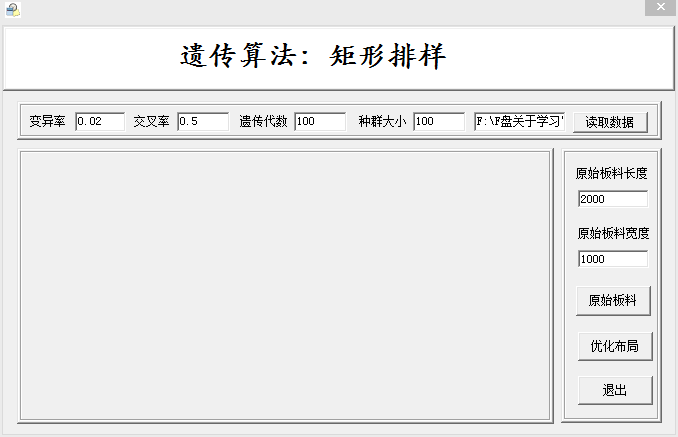测试例子的输入以文件的方式存放，输入如下测试例子：
 400 230
400 160
340 130
246 100
350 100
170 110
220 120
420 280
330 220
290 170
230 200
210 170
240 130
260 150
180 160
200 180
245 160
210 200
220 140
250 170
300 300
350 320
320 280
在原始板料长宽分别为2000、1000的情况下，点击优化布局后，结果如下：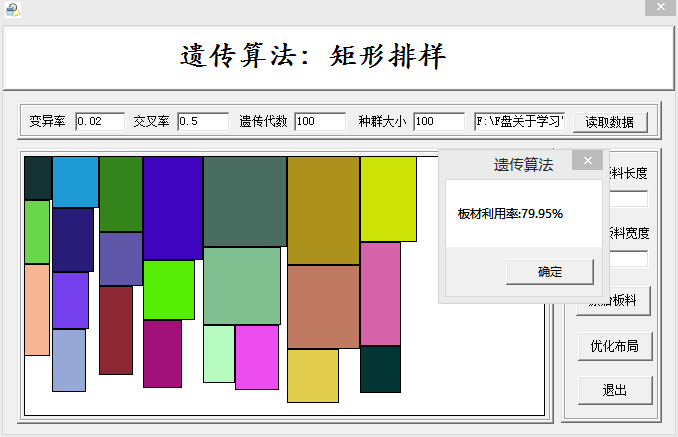注：这里的板材利用率，不是上述定义的利用率，因为上述定义的利用率很合理，但是确实不好算，需呀手工计算。为了简单起见，算的是切割矩形总面积/原材料面积。。。呵呵  工程源码  下载
展开全文遗传算法
• 求解矩形排样问题的离散粒子群算法，非常的有用，很有价值。
• 利用遗传算法解决矩形排样问题，具有可视化的界面，输入数据为含有矩形的长和宽的文本文件，输出的结果以可视化的形式显示出来
• 一种求解矩形排样问题的遗传-离散粒子群优化算法.pdf
• ## 关于矩形排样问题(二)

千次阅读 多人点赞 2015-07-09 22:09:31
上一篇博客 关于矩形排样问题(一) 引出了矩形排样问题，但是未给出解决方案，下面给出具体的解决方法以及相应的编码思路。 注：本问题的背景是第八届华中地区数学建模本科组的A题，本人侥幸拿了一等奖，呵呵，遗传...
上一篇博客 关于矩形排样问题(一) 引出了矩形排样问题，但是未给出解决方案，下面给出具体的解决方法以及相应的编码思路。  注：本问题的背景是第八届华中地区数学建模本科组的A题，本人侥幸拿了一等奖，呵呵，遗传算法只是解决问题的一个子方案，还有很多地方待优化。  在给出解决方案之前，先对遗传算法进行简单的介绍。网上关于遗传算法的例子很多，感兴趣的可以自己去钻研，对于网上的一些解释，不要觉得晦涩难懂，这是通向理解的必经之路，做科研向来都是这样[呵呵，话说得有点多]
遗传算法
提到遗传算法(Genetic Algorithm，简称GA)，自然会想到1859年达尔文提出的“物竞天择，适者生存”理论，遗传算法的思想就是基于这个理论提出的。遗传算法就是一种基于自然群体遗传进化机制的自适应全局优化概率搜索算法。模拟自然界的生物进化过程，采用人工的方式目标空间进行随机化搜索。其搜索机制大概如下：模拟自然选择和自然遗传过程中发生的繁殖、交叉和基因突变现象，在每次迭代中都保留一组候选解，并按某种指标从解群中选取较优的个体，利用遗传算子(选择、交叉和变异)对这些个体进行组合，产生新一代的候选解群，重复此过程，直到满足某种收敛指标为止。遗传算法的进化规则就是“适者生存”，较好的解保留，较差的解淘汰。基本遗传算法(Simple Genetic Algorithms,简称SGA)，是一种统一的，最基本的遗传算法，只使用选择、交叉、变异这三种基本遗传算子，其遗传进化操作过程简单，容易理解，是其他一些遗传算法的雏形和基础，它不仅给各种遗传算法提供了一个基本框架，同时也具有一定的应用价值。  生物进化与遗传算法的对应关系如下：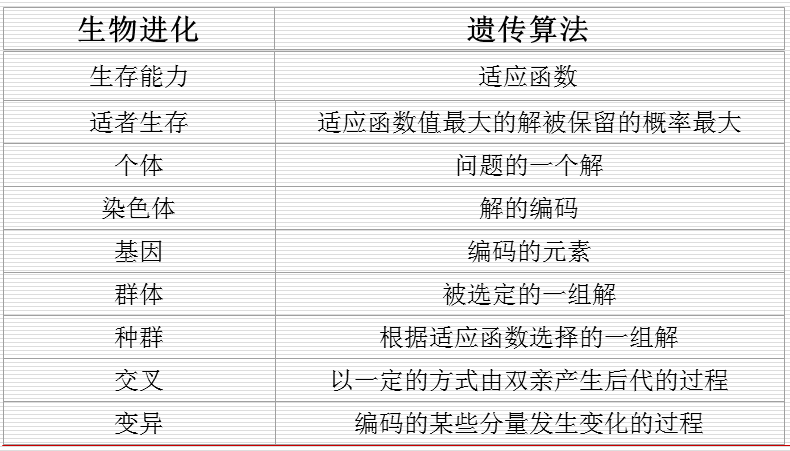遗传算法的基本操作
选择  根据各个个体的适应值，按照一定的规则或方法，从第t代群体P(t)中选择出一些优良的个体遗传到下一代群体P(t+1)中。交叉  将群体P(t)内的各个个体随机搭配成对，对每一个个体，以某个概率Pc (称为交叉概率，crossvoer rate)交换它们之间的部分染色体。变异  对群体P(t)中的每一个个体，以某一概率Pm(称为变异概率，mutation rate)改变某一个或一些基因座上基因值为其它的等位基因。  因此基于以上思路，可以知道遗传算法需要解决以下问题：  如何进行编码，如何产生初始种群，如何定义适应函数，如何进行遗传操作(选择，交叉，变异)，如何产生下一代种群，如何定义停止准则等。下面详细介绍遗传算法的各个操作

选择  对于选择操作，把当前种群的染色体按与适应值成正比例的概率复制到新的种群中，主要思想是：适应值高的染色体有较大的选择机会。选择方法有“轮盘赌”选择(Roulette wheel selection)，其主要实现的思路如下：将种群中所有染色体编号，并根据各自适应值计算按比例分配的概率，依次计算染色体累加概率，产生(0,1)之间随机数，若其最多能大于序列中第m个值，则第m个染色体被随机选择。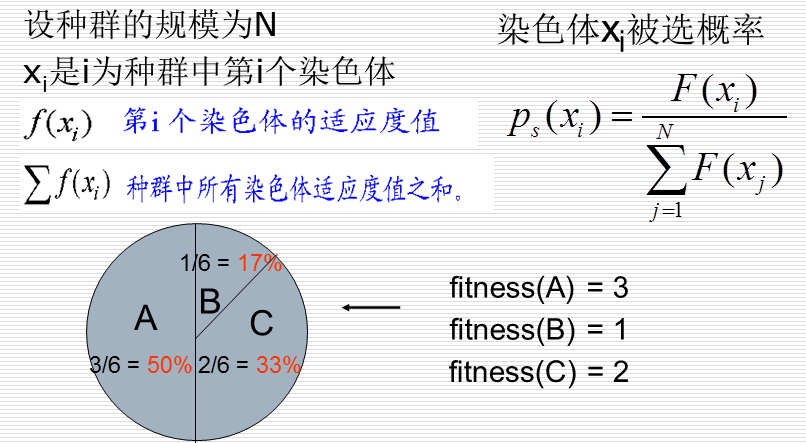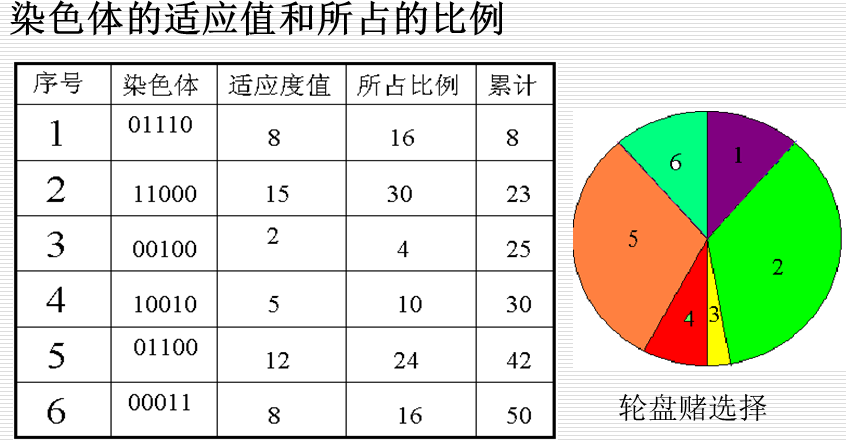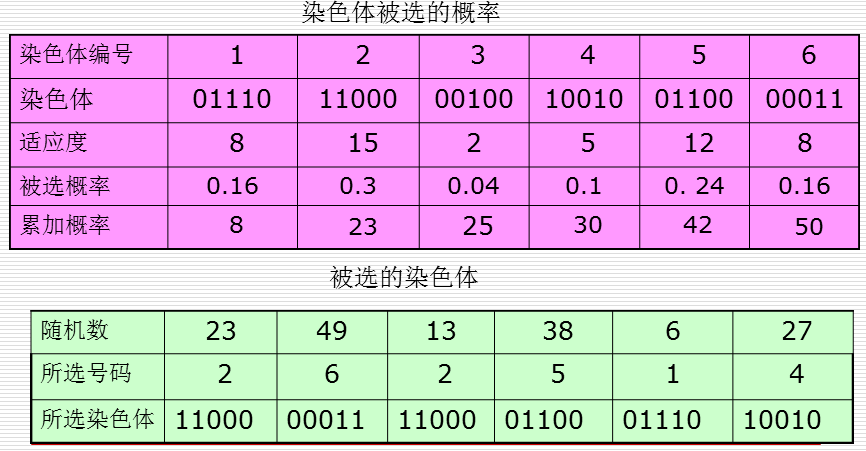轮盘上的片分配给群体的染色体，使得每一个片的大小与对于染色体的适应值成比例；从群体中选择一个染色体可视为旋转一个轮盘，当轮盘停止时，指针所指的片对于的染色体就时要选的染色体。模拟“轮盘赌”算法如下：
(1)r=random(0, 1)，s=0，i=0；
(2)如果s≥r，则转(4)；
(3)s=s+p(xi)，i=i+1, 转(2)
(4)xi即为被选中的染色体，输出I
(5)结束
其他的选择方法如下：  随机遍历抽样(Stochastic universal sampling)、局部选择(Local selection)、截断选择(Truncation selection)、竞标赛选择(Tournament selection)等。特点如下：  选择操作得到的新的群体称为交配池，交配池是当前代和下一代之间的中间群体，其规模为初始群体规模。选择操作的作用效果是提高了群体的平均适应值(低适应值个体趋于淘汰，高适应值个体趋于选择)，但这也损失了群体的多样性。选择操作没有产生新的个体，群体中最好个体的适应值不会改变。  2. 交叉  对于交叉操作，发生在两个染色体之间，由两个被称之为双亲的父代染色体，经杂交以后，产生两个具有双亲的部分基因的新的染色体，从而检测搜索空间中新的点。需要注意，选择和交叉的区别如是，选择(复制)操作每次作用在一个染色体上，而交叉操作每次作用在从交配池中随机选取的两个个体上(交叉概率Pc)。交叉产生两个子染色体，他们与其父代不同，且彼此不同, 每个子染色体都带有双亲染色体的遗传基因。对于交叉的方式，需要提的是单点交叉，单点交叉是在双亲的父代染色体中随机产生一个交叉点位置，在交叉点位置分离双亲染色体，互换交叉点位置右边的基因码产生两个子代染色体，交叉概率Pc 一般范围为(60%, 90%)，平均约80%，交叉操作示意图如下：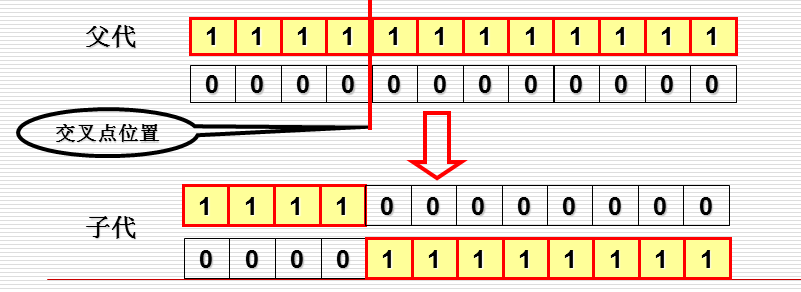单点交叉可以产生与父代染色体完全不同的子代染色体，不会改变父代染色体中相同的基因。但是，当双亲染色体相同时，交叉操作是不起作用的。例如：假如交叉概率Pc ＝50%,则交配池中50%的染色体(一半染色体)将进行交叉操作，余下的50%的染色体进行选择(复制)操作。需要说的是，遗传算法利用选择和交叉操作可以产生具有更高平均适应值和更好染色体的群体。  3. 变异  比起选择和交叉操作，变异操作是遗传算法中的次要操作，但它在恢复群体中失去的多样性方面具有潜在的作用。需要指出的是，在GA执行的开始阶段，染色体中一个特定位上的值1可能与好的性能紧密联系，即搜索空间中某些初始染色体在那个位上的值1可能一致产生高的适应值。因为越高的适应值与染色体中那个位上的值1相联系，选择操作就越会使群体的遗传多样性损失。等到达一定程度时，值0会从整个群体中那个位上消失，然而全局最优解可能在染色体中那个位上为0。如果搜索范围缩小到实际包含全局最优解的那部分搜索空间，在那个位上的值0就可能正好是到达全局最优解所需要的。  4. 适应函数  遗传算法在搜索中不依靠外部信息，仅以适应函数为依据，利用群体中每个染色体(个体)的适应值来进行搜索。以染色体适应值的大小来确定该染色体被遗传到下一代群体中的概率。染色体适应值越大，该染色体被遗传到下一代的概率也越大；反之，染色体的适应值越小，该染色体被遗传到下一代的概率也越小。因此适应函数的选取至关重要，直接影响到GA的收敛速度以及能否找到最优解。在群体中的每个染色体都需要计算适应值，适应函数一般由目标函数变换而成。常见的适应函数的形式有多种，一种是直接将目标函数转化为适应函数：若目标函数为最大化问题：Fitness(f(x)) = f(x)；若目标函数为最小化问题： Fitness(f(x)) = -f(x)，该种方式缺点如下：可能不满足轮盘赌选择中概率非负的要求；某些代求解的函数值分布上相差很大，由此得到的评价适应值可能不利于体现群体的评价性能，影响算法的性能。另一种是界限构造法，若目标函数为最大化问题：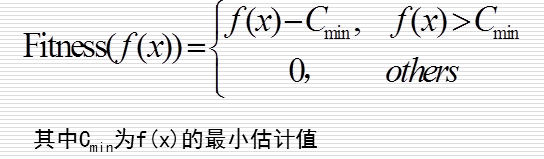若目标函数为最小化问题：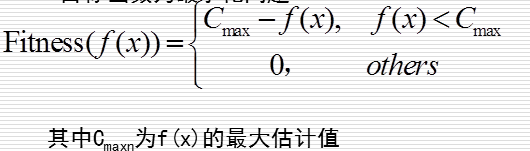5. 停止准则  停止准则的选择有多种方式，主要是下面三种方式：  种群中个体的最大适应值超过预设定值；  种群中个体的平均适应值超过预设定值；  种群中个体的进化代数超过预设定值；  经过上面的详细介绍，可以归纳出遗传算法的基本步骤如下：
(1) 随机产生初始种群;
(2) 计算种群体中每个个体的适应度值,判断是否满足停止条件，若不满足，则转第(3)步,否则转第(6)步;
(3) 按由个体适应值所决定的某个规则选择进入下一代的个体;
(4) 按交叉概率Pc进行交叉操作,生产新的个体;
(5) 按变异概率Pm进行变异操作,生产新的个体;
(6) 输出种群中适应度值最优的染色体作为问题的满意解或最优解。
流程图如下：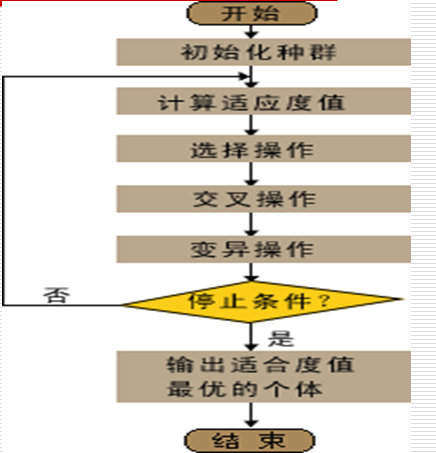整个算法的伪代码如下：
Procedure Genetic Algorithm
begin
t = 0 ;
初始化 P(t) ;
计算 P(t) 的适应值 ;
while (不满足停止准则) do
begin
t = t+1 ;
从P(t-1)中选择 P(t) ;    % selection
重组 P(t) ;                    % crossover and mutation
计算 P(t) 的适应值;
end
end
遗传算法作为经典的优化算法，研究得已经很成熟了，在网上可以找到该算法的针对各种编程语言的工具箱，呵呵。
展开全文遗传算法 数学建模
• 二维多阶段矩形剪切排样算法(精)第３２卷第５期　计算机应用与软件 Ｖｏｌ３２Ｎｏ．５５２０１５年５月　ＣｏｍｐｕｔｅｒＡｐｐｌｉｃａｔｉｏｎｓａｎｄＳｏｆｔｗａｒｅ Ｍａｙ２０１二维多阶段矩形剪切...

二维多阶段矩形剪切排样算法(精)
第３２卷第５期　　　 计算机应用与软件 Ｖｏｌ３２Ｎｏ．５
５
２０１５年５月　　 ＣｏｍｐｕｔｅｒＡｐｐｌｉｃａｔｉｏｎｓａｎｄＳｏｆｔｗａｒｅ Ｍａｙ２０１
二维多阶段矩形剪切排样算法
孔令熠　陈秋莲
(广西大学计算机与电子信息学院　广西南宁５３０００４)
摘　要　　讨论有需求约束的二维剪切矩形排样问题：将一张板材剪切成一组已知尺寸的毛坯，使排样价值(板材中包含的毛坯总
价值)最大，约束条件是排样方式中包含每种毛坯数量都不能超过其需求量。采用普通条带多阶段排样方式，每次剪切都从板材上
产生一根水平或者竖直的普通条带，条带中可以包含不同尺寸毛坯。引入分支限界与贪婪策略，以提高算法效率。实验结果表明，
该算法可以有效提高排样价值。
关键词　　有约束二维剪切　多阶段排样方式　普通条带　分支限界　贪婪策略
中图分类号　ＴＰ３９１　　　　文献标识码　Ａ　　　　ＤＯＩ：１０．３９６９／ｊ．ｉｓｓｎ．１０００３８６ｘ．２０１５．０５．０５６
ＭＵＬＴＩＳＴＡＧＥＴＷＯＤＩＭＥＮＳＩＯＮＡＬＲＥＣＴＡＮＧＵＬＡＲＮＥＳＴＩＮＧＡＬＧＯＲＩＴＨＭ
ＷＩＴＨＣＵＴＴＩＮＧＢＹＧＵＩＬＬＯＴＩＮＥ
ＫｏｎｇＬｉｎｇｙｉ　ＣｈｅｎＱｉｕｌｉａｎ
(ＣｏｌｌｅｇｅｏｆＣｏｍｐｕｔｅｒａｎｄＥｌｅｃｔｒｏｎｉｃＩｎｆｏｒｍａｔｉｏｎ，ＧｕａｎｇｘｉＵｎｉｖｅｒｓｉｔｙ，Ｎａｎｎｉｎｇ５３０００４，Ｇｕａｎｇｘｉ，Ｃｈｉｎａ)
Ａｂｓｔｒａｃｔ　　Ｗｅｄｉｓｃｕｓｓｔｗｏｄｉｍｅｎｓｉｏｎａｌｃｕｔｔｉｎｇｒｅｃｔａｎｇｕｌａｒｎｅｓｔｉｎｇｐｒｏｂｌｅｍｗｉｔｈｃｏｎｓｔｒａｉｎｅｄｒｅｑｕｉｒｅｍｅｎｔ：ｉｎｗｈｉｃｈａｓｉｎｇｌｅｐｌａｔｅｉｓｃｕｔｉｎｔｏ
ａｇｒｏｕｐｏｆｒｏｕｇｈｃａｓｔｐｉｅｃｅｓｗｉｔｈｇｉｖｅｎｓｉｚｅｓａｎｄｍａｋｅｓｔｈｅｎｅｓｔｉｎｇｖａｌｕｅ(ｔｏｔａｌｖａｌｕｅｏｆｐｉｅｃｅｓｃｏｎｔａｉｎｅｄｉｎｔｈｅｐｌａｔｅ)ｍａｘｉｍｉｓｅｄ，ｔｈｅ
ｃｏｎｓｔｒａｉｎｔｓａｒｅｔｈａｔｉｎｔｈｅｎｅｓｔｉｎｇｐａｔｔｅｒｎｔｈｅｎｕｍｂｅｒｏｆｅａｃｈｐｉｅｃｅｃａｎｎｏｔｅｘｃｅｅｄｉｔｓｄｅｍａｎｄ．Ｗｅａｄｏｐｔｇｅｎｅｒａｌｓｔｒｉｐｓａｎｄｍｕｌｔｉｓｔａｇｅｄ
ｎｅｓｔｉｎｇｐａｔｔｅｒｎ，ｔｈｅｒｅｗｉｌｌｐｒｏｄｕｃｅｏｎｅｈｏｒｉｚｏｎｔａｌｏｒｖｅｒｔｉｃａｌｓｔｒｉｐｆｒｏｍｔｈｅｐｌａｔｅｉｎｅｖｅｒｙｃｕｔ，ｗｈｉｃｈｃａｎｃｏｎｔａｉｎｔｈｅｐｉｅｃｅｓｏｆｄｉｆｆｅｒｅｎｔｔｙｐｅｓ．
Ｂｒａｎｃｈａｎｄｂｏｕｎｄａｎｄｇｒｅｅｄｙｓｔｒａｔｅｇｙａｒｅｉｎｔｒｏｄｕｃｅｄｔｏｅｎｈａｎｃｅｔｈｅｅｆｆｉｃｉｅｎｃｙｏｆｔｈｅａｌｇｏｒｉｔｈｍ．Ｅｘｐｅｒｉｍｅｎｔａｌｒｅｓｕｌｔｓｉｎｄｉｃａｔｅｔｈａｔｔｈｅ
ａｌｇｏｒｉｔｈｍｃａｎｅｆｆｅｃｔｉｖｅｌｙｉｍｐｒｏｖｅｔｈｅｖａｌｕｅｏｆｎｅｓｔｉｎｇ．
Ｋｅｙｗｏｒｄｓ　　Ｃｏｎｓｔｒａｉｎｅｄｔｗｏｄｉｍｅｎｓｉｏｎａｌｃｕｔｔｉｎｇｐｒｏｂｌｅｍｓ　Ｍｕｌｔｉｓｔａｇｅｎｅｓｔｉｎｇｐａｔｔｅｒｎ　Ｇｅｎｅｒａｌｓｔｒｉｐ　Ｂｒａｎｃｈａｎｄｂｏｕｎｄ
Ｇｒｅｅｄｙｓｔｒａｔｅｇｙ
［７］：从
ＤＴ) Ｌ×Ｗ的板材上切下ｍ种毛坯，其中第ｉ种毛坯的尺寸
０　引　言 和价值分别是ｌ×ｗ和ｃ，需求量为ｄ。使用冲压机剪裁方式

展开全文• 基于算法群模式的矩形排样算法设计及其C++实现（一） 设计思想篇 矩形排样概述 矩形排样，是指在预先已确定长宽的“原材料板件”上，按照一定的算法规则，尽可能多的排布不同大小规格的矩形“切割板件”，以...算法 c++ 模拟退火算法 贪心算法
• ## 关于矩形排样问题(一)

千次阅读 多人点赞 2015-05-04 11:34:01
最近看到几个比较有意思的软件：极致下料、Cutlogic 2D、新易优化板材切割等软件，都围绕一个共同的话题，即板材切割，主要是针对二维的。对于上述软件，下载...好奇心的驱动，自己也研究了一些矩形排样的文章。准备
• 矩形优化排样问题是一个在制造业领域生产实践中普遍遇到的问题 ,采用了一种改进的最低水平线搜索算法求解此类问题 .首先分析了原始的最低水平线搜索算法在排样中存在的缺陷 ,并针对该缺陷为其设计了一个评价函数 ,...
• 最近参见华中赛，选了A题，钢构件的排料问题，问题解决期间用到了遗传算法，自己用VC写了一个，这个是界面部分
• ## 矩形排样问题 遗传算法解决方案

万次阅读 多人点赞 2017-04-05 14:10:52
客户要将若干大小不一的小矩形，排到大矩形上，而且还要求可以设置小矩形之间的间距，和大矩形的margin值，便于裁切。 排样问题是一个经典的NP问题，有很多解决方案。神经网络、遗传、蚁群、模拟退火等等算法都可以...
• 排样变量与关系 此算法，基于固定4边的尺寸遍历每个单只板的长宽得到最优解。 二.条件约束 基本约束条件(参考上图变量) 三.排样图形相同类型规律 由于计算量大，为了有效减少计算量,提高效率，将排样......